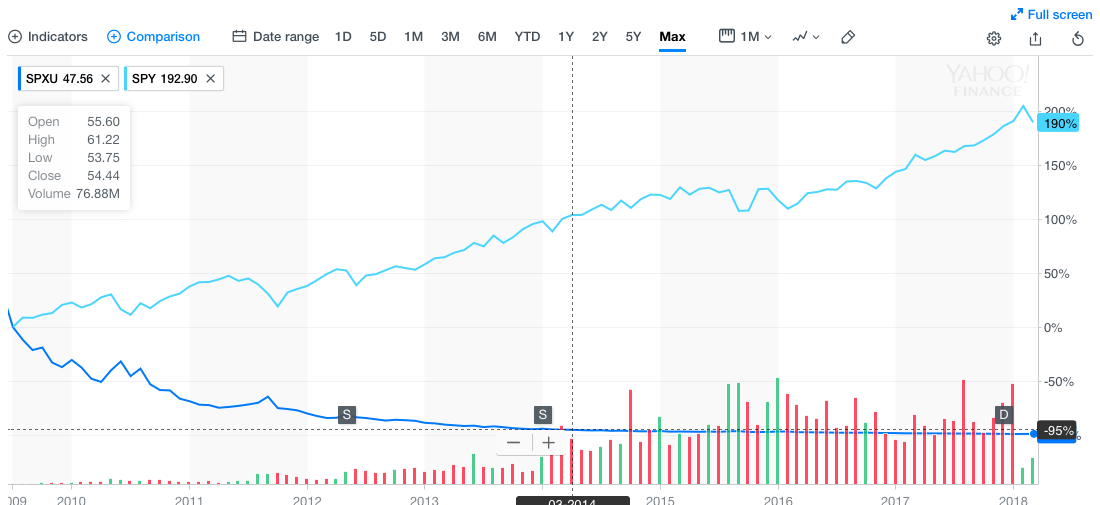Dr Eberhard Mayerhofer

Investing directly into an index such as the Nasdaq, S&P 500, or Russel 2000 is impractical for individual investors, as they would have to trade hundreds of stocks and reinvest their dividends each time that one of these companies pays one. For instance, the S&P 500 represents approximately 500 firms listed on the NYSE and NADAQ, which contribute to the index with a certain fixed weight. One could check the weighting of each index constituent and purchase shares accordingly. This would however result in a very costly portfolio, since one would have to purchase at least one share of each of the 500 stocks (On 1st March 2018 this would amount to 54172.98 US Dollar, for the S&P 500).

Exchange traded funds (ETFs) allow any investor to hold a portfolio that tracks an index’ performance, before management fees. They pool capital from hundreds of thousands, if not millions, of investors, and then invest in the index’ underlying basket of securities, thereby closely tracking its performance. By owning a small fraction of the fund’s portfolio, investor delegate portfolio management to the fund and share its costs.

For example, the oldest and largest ETF is the SPY, which tracks the performance of the S&P 500 index. The price of one of its shares on March 1 was 267.70 US Dollars. On the following day, the S&P 500 rose 0.5%, whence an investor who had purchased 1 share of SPY on March 1st, on the following day would have had 267.70*(1+s%)=269.06 USD. The SPY generates these returns simply by investing in the same portfolio used in the calculation of the S&P 500 index, with the same weights. In return, the fund charges a small fee (0.09% per annum), so that the fund’s returns is the index returns minus such fee.

In contrast to normal ETFs, Leveraged and Inverse Exchange Traded Funds (LIETFs) go a step further: They promise a multiple, such as L=+3, +2 (or for inverse L=-1,-2, -3) of the daily index return. The underlying idea is that an upward trend of the index would be magnified accordingly (respectively a downward trend with an inverse ETF). In the below Figure, we see the prices of SSO (2x S&P500) and SPY (1x S&P500). Obviously, the index trended up, and the SSO magnified the trend by a factor of 2.How does an ETF trade to achieve its goal? Let us assume cash can be borrowed at no cost. To achieve an  L*s% return on a 100 \$ risky investment, one needs to borrow (L-1) 100 \$ extra cash.

The following summarizes the investments on day 1 and day 2:

•    (cash position) -(L-1)100 \$ (day 1), and -(L-1)100 (day 2)

• (risky position) 100*L \$ (day 1), and 100L (1+s%) (day 2).

The return on day 2 is indeed Ls% (=({100*L (1+s%)- (L-1)100}-100)/100

In reality, interest rates are not zero, therefore only excess returns can be multiplied with this strategy. The actual excess return of the leveraged portfolio is then the respective multiple of the excess return, minus the expense ratio. A LIETFs becomes riskier as its multiple increases. In fact, for large enough multipliers, and risky enough reference indices, the value of a share of an LIETF may tend to zero. This effect, also known as volatility decay, results from the ups and downs of the index, rather than consistent upward movements: In the below figure we see that SPXU (-3x SPY) lost dramatically in value (here in comparison with SPY) Therefore it is not advisable to invest into these for a long period, but rather actively trade them.Let’s demonstrate this decay with some figures: suppose that the index falls by 20%. To recover, it has to rise by 25%, because (1-20%)=1/(1+25%). Knowing that daily expense ratios and interest rates are negligible at such large market movements, the respective LETF will therefore have an approximate value of (1-L20%)(1+L25%), which gives a fund value of 90% (L=2) or even 70% (L=3) of the initial investment. For, say 10 of such up and downward ticks, independent of their chronological order, the fund values have dramatically lost in value: (L=2) 3.5% of initial investment (L=3) 3% of initial investment. Maintaining constant leverage requires frequent trading in all of the index stocks. In fact, if an investment of 100 dollars devalues to 80, the value of the investment in a 2x ETF becomes 60, while its exposure 200(1-0.2) = 160, which is above the required 260 = 120. Thus, the ETF needs to reduce its position by selling 40 dollars of the asset. Likewise, if the index rises by 25%, the investment is now worth 150, but the exposure is 200(1+0.25) = 250, which is less than the 150*2 = 300 required. Thus, the ETF needs to increase its position by selling 50 dollars of the asset. In reality, such daily rebalancing is costly, and detracts further form the ETF performance, in addition to management fees. Leverage is not unique to LETFs; they exist explicitly or implicitly in many financial products (e.g. the so-called certificates in Germany, Switzerland, Italy with much higher multipliers, or options contracts with embedded leverage). While normal ETFs exist since the early 1990s, leveraged ETFs only came into existence in 2006.

While empirical research on LIETF has begun to appear in the past decade, Paolo Guasoni (DCU) and Eberhard Mayerhofer(UL) offer a performance theory that explains the deviation from theoretical daily benchmarks (the so-called tracking error) by transaction costs, which funds face for daily rebalancing their portfolios, in order to maintain the correct leverage. Optimally managing LIETF creates a trade-off between a small tracking error on one hand, and avoiding losses due to transaction costs on the other hand. Even funds that trade optimally trading ETFs on the same index can be difficult to compare without such a theory, due to potentially different objectives concerning return and tracking error.

Eberhard Mayerhofer joined the Department of Mathematics & Statistics in 2016. His research is on Applied Probability and Financial Mathematics.